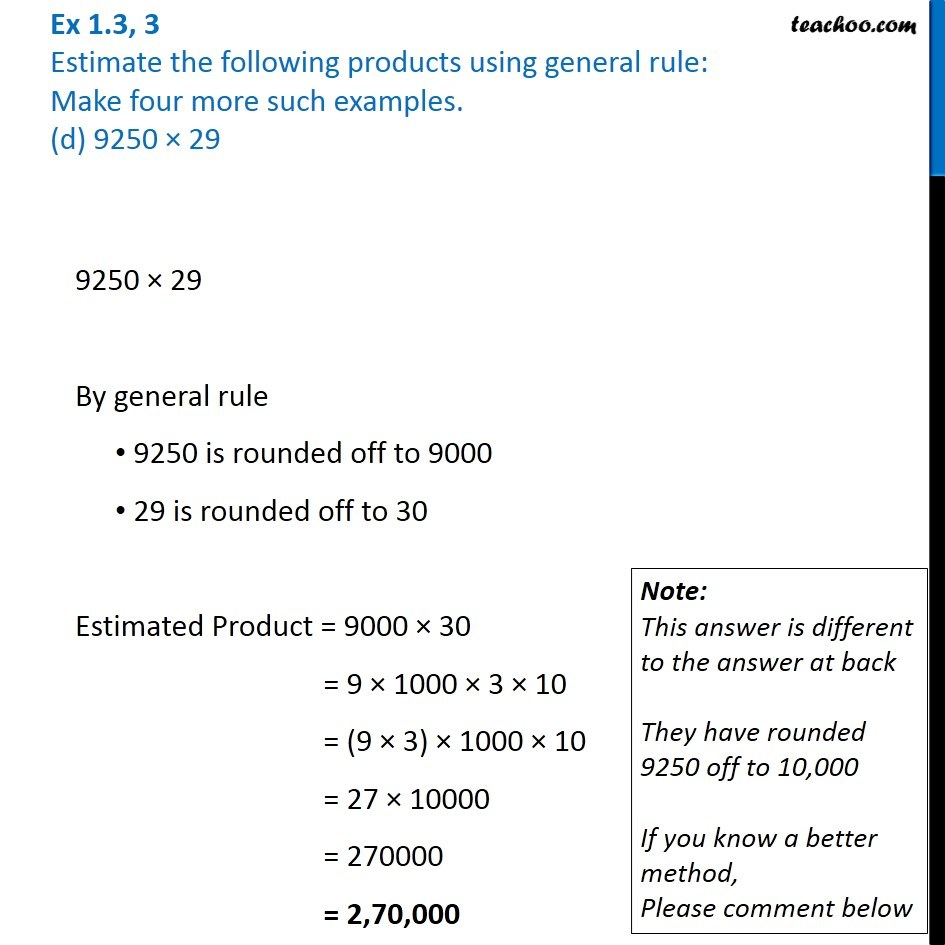1. Chapter 1 Class 6 Knowing our Numbers
2. Serial order wise
3. Ex 1.3

Transcript

Ex 1.3, 3 Estimate the following products using general rule: Make four more such examples. (d) 9250 × 299250 × 29 By general rule 9250 is rounded off to 9000 29 is rounded off to 30 Estimated Product = 9000 × 30 = 9 × 1000 × 3 × 10 = (9 × 3) × 1000 × 10 = 27 × 10000 = 270000 = 2,70,000 Note: This answer is different to the answer at back They have rounded 9250 off to 10,000 If you know a better method, Please comment below